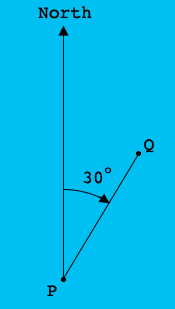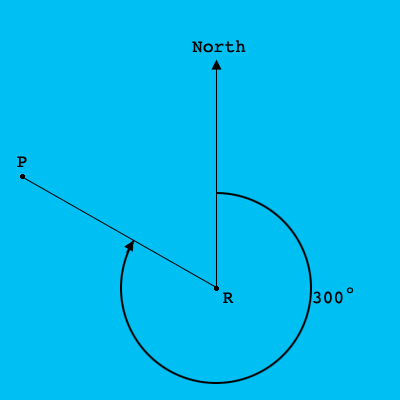SEARCH HOMEMath Central Quandaries & QueriesQuestion from Deborah: three ships p q and r are at sea. the bearing of q from p is 30° and the bearing of p from r is 300°. if |PQ|=5km and|PR|=8km I. illustrate the information in a diagram II. calculate correct to 3sf the I. distance between Q and R. II. bearing of R from QHi Deborah,

The bearing of $P$ to $Q$ is the measure of the angle from north to the line segment $PQ$ measured clockwise from north.The bearing of $R$ to $P$ is the measure of the angle from north to the line segment $RP$ measured clockwise from north.Put both these diagrams on the same diagram.

PennyMath Central is supported by the University of Regina and The Pacific Institute for the Mathematical Sciences.